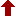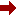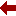#### SLIM21

 Sort Order Chronological Author Title ItemType,Author ItemType,Title Display Format Tabular Publication Bibliography Items / Page 5 10 25

Click the serial number on the left to view the details of the item.
#  AuthorTitleAccn#YearItem Type Claims
1 Selinger, Jonathan V Introduction to the Theory of Soft Matter I10283 2016 eBook
2 Kantorovich, Lev Mathematics for Natural Scientists II I10062 2016 eBook
3 Sahni, Viraht Quantal Density Functional Theory I09642 2016 eBook
4 Kantorovich, Lev Mathematics for Natural Scientists I09133 2016 eBook
5 Conte, Robert The Painlev?? Handbook I09128 2020 eBook
6 Seaborn, James B Mathematics for the Physical Sciences I11155 2002 eBook
7 Hassani, Sadri Mathematical Methods Using Mathematica?? I10927 2003 eBook
8 Shizgal, Bernard Spectral Methods in Chemistry and Physics I08216 2015 eBook
9 Britz, Dieter Digital Simulation in Electrochemistry I07455 2005 eBook
10 Thornton, Colin Granular Dynamics, Contact Mechanics and Particle System Simulations I07196 2015 eBook
 (page:1 / 4) [#32] Next Page Last Page

1.TitleIntroduction to the Theory of Soft Matter : From Ideal Gases to Liquid Crystals
Author(s)Selinger, Jonathan V
PublicationCham, Springer International Publishing, 2016.
DescriptionX, 185 p. 78 illus., 69 illus. in color : online resource
Abstract NoteThis book presents the theory of soft matter to students at the advanced undergraduate or beginning graduate level. It provides a basic introduction to theoretical physics as applied to soft matter, explaining the concepts of symmetry, broken symmetry, and order parameters; phases and phase transitions; mean-field theory; and the mathematics of variational calculus and tensors. It is written in an informal, conversational style, which is accessible to students from a diverse range of backgrounds. The book begins with a simple ???toy model??? to demonstrate the physical significance of free energy. It then introduces two standard theories of phase transitions???the Ising model for ferromagnetism and van der Waals theory of gases and liquids???and uses them to illustrate principles of statistical mechanics. From those examples, it moves on to discuss order, disorder, and broken symmetry in many states of matter, and to explain the theoretical methods that are used to model the phenomena. It concludes with a chapter on liquid crystals, which brings together all of these physical and mathematical concepts. The book is accompanied by a set of ???interactive figures,??? which allow online readers to change parameters and see what happens to a graph, some allow users to rotate a plot or other graphics in 3D, and some do both. These interactive figures help students to develop their intuition for the physical meaning of equations. This book will prepare advanced undergraduate or early graduate students to go into more advanced theoretical studies. It will also equip students going into experimental soft matter science to be fully conversant with the theoretical aspects and have effective collaborations with theorists
ISBN,Price9783319210544
Keyword(s)1. Amorphous substances 2. Biomedical engineering 3. Biomedical Engineering and Bioengineering 4. Chemometrics 5. Complex fluids 6. EBOOK 7. EBOOK - SPRINGER 8. Math. Applications in Chemistry 9. PHYSICAL CHEMISTRY 10. Soft and Granular Matter, Complex Fluids and Microfluidics
Item TypeeBook
Circulation Data
 Accession# Call# Status Issued To Return Due On Physical Location I10283 On Shelf

2.TitleMathematics for Natural Scientists II : Advanced Methods
Author(s)Kantorovich, Lev
PublicationCham, Springer International Publishing, 2016.
DescriptionXVII, 675 p. 107 illus., 104 illus. in color : online resource
Abstract NoteThis book covers the advanced mathematical techniques useful for physics and engineering students, presented in a form accessible to physics students, avoiding precise mathematical jargon and laborious proofs. Instead, all proofs are given in a simplified form that is clear and convincing for a physicist. Examples, where appropriate, are given from physics contexts. Both solved and unsolved problems are provided in each chapter. Mathematics for Natural Scientists II: Advanced Methods is the second of two volumes. It follows the first volume on Fundamentals and Basics
ISBN,Price9783319278612
Keyword(s)1. APPLIED MATHEMATICS 2. Chemometrics 3. EBOOK 4. EBOOK - SPRINGER 5. ENGINEERING MATHEMATICS 6. Math. Applications in Chemistry 7. Mathematical and Computational Engineering 8. Mathematical Applications in the Physical Sciences 9. Mathematical Methods in Physics 10. MATHEMATICAL PHYSICS 11. Numerical and Computational Physics, Simulation 12. PHYSICS
Item TypeeBook
Circulation Data
 Accession# Call# Status Issued To Return Due On Physical Location I10062 On Shelf

3.TitleQuantal Density Functional Theory
Author(s)Sahni, Viraht
PublicationBerlin, Heidelberg, Springer Berlin Heidelberg, 2016.
DescriptionXIX, 413 p. 71 illus., 20 illus. in color : online resource
Abstract NoteThis book deals with quantal density functional theory (QDFT) which is a time-dependent local effective potential theory of the electronic structure of matter. The treated time-independent QDFT constitutes a special case. In the 2nd edition, the theory is extended to include the presence of external magnetostatic fields. The theory is a description of matter based on the ???quantal Newtonian??? first and second laws which is in terms of ???classical??? fields that pervade all space, and their quantal sources. The fields, which are explicitly defined, are separately representative of electron correlations due to the Pauli exclusion principle, Coulomb repulsion, correlation-kinetic, correlation-current-density, and correlation-magnetic effects. The book further describes Schr??dinger theory from the new physical perspective of fields and quantal sources. It also describes traditional Hohenberg-Kohn-Sham DFT, and explains via QDFT the physics underlying the various energy functionals and functional derivatives of the traditional approach to electronic structure.
ISBN,Price9783662498422
Keyword(s)1. Chemometrics 2. EBOOK 3. EBOOK - SPRINGER 4. Interfaces (Physical sciences) 5. Materials???Surfaces 6. Math. Applications in Chemistry 7. MATHEMATICAL PHYSICS 8. Surface and Interface Science, Thin Films 9. Surfaces (Physics) 10. Surfaces and Interfaces, Thin Films 11. Theoretical, Mathematical and Computational Physics 12. THIN FILMS
Item TypeeBook
Circulation Data
 Accession# Call# Status Issued To Return Due On Physical Location I09642 On Shelf

4.TitleMathematics for Natural Scientists : Fundamentals and Basics
Author(s)Kantorovich, Lev
PublicationNew York, NY, Springer New York, 2016.
DescriptionXVII, 526 p. 124 illus., 118 illus. in color : online resource
Abstract NoteThis book, the first in a two part series, covers a course of mathematics tailored specifically for physics, engineering and chemistry students at the undergraduate level. It is unique in that it begins with logical concepts of mathematics first encountered at A-level and covers them in thorough detail, filling in the gaps in students' knowledge and reasoning. Then the book aids the leap between A-level and university-level mathematics, with complete proofs provided throughout and all complex mathematical concepts and techniques presented in a clear and transparent manner. Numerous examples and problems (with answers) are given for each section and, where appropriate, mathematical concepts are illustrated in a physics context. This text gives an invaluable foundation to students and a comprehensive aid to lecturers. Mathematics for Natural Scientists: Fundamentals and Basics is the first of two volumes. Advanced topics and their applications in physics are covered in the second volume
ISBN,Price9781493927852
Keyword(s)1. APPLIED MATHEMATICS 2. Chemometrics 3. EBOOK 4. EBOOK - SPRINGER 5. ENGINEERING MATHEMATICS 6. Math. Applications in Chemistry 7. Mathematical and Computational Engineering 8. Mathematical Applications in the Physical Sciences 9. Mathematical Methods in Physics 10. MATHEMATICAL PHYSICS 11. Numerical and Computational Physics, Simulation 12. PHYSICS
Item TypeeBook
Circulation Data
 Accession# Call# Status Issued To Return Due On Physical Location I09133 On Shelf

5.TitleThe Painlev?? Handbook
Author(s)Conte, Robert;Musette, Micheline
PublicationCham, Springer International Publishing, 2020.
DescriptionXXXI, 389 p. 15 illus., 6 illus. in color : online resource
Abstract NoteThis book, now in its second edition, introduces the singularity analysis of differential and difference equations via the Painlev?? test and shows how Painlev?? analysis provides a powerful algorithmic approach to building explicit solutions to nonlinear ordinary and partial differential equations. It is illustrated with integrable equations such as the nonlinear Schr??dinger equation, the Korteweg-de Vries equation, H??non-Heiles type Hamiltonians, and numerous physically relevant examples such as the Kuramoto-Sivashinsky equation, the Kolmogorov-Petrovski-Piskunov equation, and mainly the cubic and quintic Ginzburg-Landau equations. Extensively revised, updated, and expanded, this new edition includes: recent insights from Nevanlinna theory and analysis on both the cubic and quintic Ginzburg-Landau equations; a close look at physical problems involving the sixth Painlev?? function; and an overview of new results since the book???s original publication with special focus on finite difference equations. The book features tutorials, appendices, and comprehensive references, and will appeal to graduate students and researchers in both mathematics and the physical sciences
ISBN,Price9783030533403
Keyword(s)1. APPLIED MATHEMATICS 2. Chemometrics 3. Dynamical Systems and Ergodic Theory 4. DYNAMICS 5. EBOOK 6. EBOOK - SPRINGER 7. ENGINEERING MATHEMATICS 8. ERGODIC THEORY 9. Math. Applications in Chemistry 10. Mathematical and Computational Engineering 11. Mathematical Methods in Physics 12. MATHEMATICAL PHYSICS 13. PARTIAL DIFFERENTIAL EQUATIONS 14. PHYSICS
Item TypeeBook
Circulation Data
 Accession# Call# Status Issued To Return Due On Physical Location I09128 On Shelf

6.TitleMathematics for the Physical Sciences
Author(s)Seaborn, James B
PublicationNew York, NY, Springer New York, 2002.
DescriptionXI, 245 p : online resource
Abstract NoteThis book is intended to provide a mathematical bridge from a general physics course to intermediate-level courses in classical mechanics, electricity and mag?? netism, and quantum mechanics. The book begins with a short review of a few topics that should be familiar to the student from a general physics course. These examples will be used throughout the rest of the book to provide physical con?? texts for introducing the mathematical applications. The next two chapters are devoted to making the student familiar with vector operations in algebra and cal?? culus. Students will have already become acquainted with vectors in the general physics course. The notion of magnetic flux provides a physical connection with the integral theorems of vector calculus. A very short chapter on complex num?? bers is sufficient to supply the needed background for the minor role played by complex numbers in the remainder of the text. Mathematical applications in in?? termediate and advanced undergraduate courses in physics are often in the form of ordinary or partial differential equations. Ordinary differential equations are introduced in Chapter 5. The ubiquitous simple harmonic oscillator is used to il?? lustrate the series method of solving an ordinary, linear, second-order differential equation. The one-dimensional, time-dependent SchrOdinger equation provides an illus?? tration for solving a partial differential equation by the method of separation of variables in Chapter 6
ISBN,Price9781468492798
Keyword(s)1. APPLIED MATHEMATICS 2. Chemometrics 3. EBOOK 4. EBOOK - SPRINGER 5. ENGINEERING MATHEMATICS 6. Math. Applications in Chemistry 7. Mathematical and Computational Engineering 8. Mathematical Methods in Physics 9. Numerical and Computational Physics, Simulation 10. PHYSICS
Item TypeeBook
Circulation Data
 Accession# Call# Status Issued To Return Due On Physical Location I11155 On Shelf

7.TitleMathematical Methods Using Mathematica?? : For Students of Physics and Related Fields
PublicationNew York, NY, Springer New York, 2003.
DescriptionXV, 235 p. 101 illus : online resource
Abstract NoteIntended as a companion for textbooks in mathematical methods for science and engineering, this book presents a large number of numerical topics and exercises together with discussions of methods for solving such problems using Mathematica(R).??Although it is primarily designed for use with the author's "Mathematical Methods: For Students of Physics and Related Fields," the discussions in the book sufficiently self-contained that the book can be used as a supplement to any of the standard textbooks in mathematical methods for undergraduate students of physical sciences or engineering
ISBN,Price9780387215594
Keyword(s)1. APPLIED MATHEMATICS 2. Chemometrics 3. EBOOK 4. EBOOK - SPRINGER 5. ENGINEERING MATHEMATICS 6. Math. Applications in Chemistry 7. Mathematical and Computational Engineering 8. Mathematical Methods in Physics 9. PHYSICS
Item TypeeBook
Circulation Data
 Accession# Call# Status Issued To Return Due On Physical Location I10927 On Shelf

8.TitleSpectral Methods in Chemistry and Physics : Applications to Kinetic Theory and Quantum Mechanics
Author(s)Shizgal, Bernard
PublicationDordrecht, Springer Netherlands, 2015.
DescriptionXVII, 415 p. 102 illus., 2 illus. in color : online resource
Abstract NoteThis book is a pedagogical presentation of the application of spectral and pseudospectral methods to kinetic theory and quantum mechanics. There are additional applications to astrophysics, engineering, biology and many other fields. The main objective of this book is to provide the basic concepts to enable the use of spectral and pseudospectral methods to solve problems in diverse fields of interest and to a wide audience. While spectral methods are generally based on Fourier Series or Chebychev polynomials, non-classical polynomials and associated quadratures are used for many of the applications presented in the book. Fourier series methods are summarized with a discussion of the resolution of the Gibbs phenomenon. Classical and non-classical quadratures are used for the evaluation of integrals in reaction dynamics including nuclear fusion, radial integrals in density functional theory, in elastic scattering theory and other applications. The subject matter includes the calculation of transport coefficients in gases and other gas dynamical problems based on spectral and pseudospectral solutions of the Boltzmann equation. Radiative transfer in astrophysics and atmospheric science, and applications to space physics are discussed.?? The relaxation of initial non-equilibrium distributions to equilibrium for several different systems is studied with the Boltzmann and Fokker-Planck equations. The eigenvalue spectra of the linear operators in the Boltzmann, Fokker-Planck and Schr??dinger equations are studied with spectral and pseudospectral methods based on non-classical orthogonal polynomials. The numerical methods referred to as the Discrete Ordinate Method, Differential Quadrature, the Quadrature Discretization Method, the Discrete Variable Representation, the Lagrange Mesh Method, and others are discussed and compared. MATLAB codes are provided for most of the numerical results reported in the book
ISBN,Price9789401794541
Keyword(s)1. Chemometrics 2. EBOOK 3. EBOOK - SPRINGER 4. Math. Applications in Chemistry 5. MATHEMATICAL PHYSICS 6. PHYSICAL CHEMISTRY 7. QUANTUM PHYSICS 8. Theoretical, Mathematical and Computational Physics
Item TypeeBook
Circulation Data
 Accession# Call# Status Issued To Return Due On Physical Location I08216 On Shelf

9.TitleDigital Simulation in Electrochemistry
Author(s)Britz, Dieter
PublicationBerlin, Heidelberg, Springer Berlin Heidelberg, 2005.
DescriptionXIII, 338 p. 252 illus : online resource
Abstract NoteThe book shows how to numerically solve the parabolic partial differential equations (pdes) encountered in electroanalytical chemistry. It does this in a didactic manner, by first introducing the basic equations to be solved and some model systems as text cases, for which solutions exist. Then it treats basic numerical approximation for derivatives and techniques for the numerical solution of ordinary differential equations, from which the more complicated methods for pdes can be derived. The major implicit methods are described in detail, and the handling of homogeneous chemical reactions, including coupled and nonlinear cases, is detailed. More advanced techniques are presented briefly, as well as some commercially available program packages. This third edition, still the only text on the subject, is structured more didactically then the previous editions. It brings the coverage of electrochemical digital simulation up to date, including many new techniques developed since the early 1990s. A number of example procedures and programs are made available as supplementary material in the online version of this book
ISBN,Price9783540315247
Keyword(s)1. Analytical chemistry 2. Chemistry, Physical and theoretical 3. Chemometrics 4. COMPLEXITY 5. COMPUTATIONAL COMPLEXITY 6. EBOOK 7. EBOOK - SPRINGER 8. ELECTRONICS 9. Electronics and Microelectronics, Instrumentation 10. Math. Applications in Chemistry 11. MICROELECTRONICS 12. PHYSICAL CHEMISTRY 13. Theoretical and Computational Chemistry
Item TypeeBook
Circulation Data
 Accession# Call# Status Issued To Return Due On Physical Location I07455 On Shelf

10.TitleGranular Dynamics, Contact Mechanics and Particle System Simulations : A DEM study
Author(s)Thornton, Colin
PublicationCham, Springer International Publishing, 2015.
DescriptionXIII, 195 p. 144 illus., 13 illus. in color : online resource
Abstract NoteThis book is devoted to the Discrete Element Method (DEM) technique, a discontinuum modelling approach that takes into account the fact that granular materials are composed of discrete particles which interact with each other at the microscale level. This numerical simulation technique can be used both for dispersed systems in which the particle-particle interactions are collisional and compact systems of particles with multiple enduring contacts. The book provides an extensive and detailed explanation of the theoretical background of DEM. Contact mechanics theories for elastic, elastic-plastic, adhesive elastic and adhesive elastic-plastic particle-particle interactions are presented. Other contact force models are also discussed, including corrections to some of these models as described in the literature, and important areas of further research are identified. A key issue in DEM simulations is whether or not a code can reliably simulate the simplest of systems, namely the single particle oblique impact with a wall. This is discussed using the output obtained from the contact force models described earlier, which are compared for elastic and inelastic collisions.??In addition, further insight is provided for the impact of adhesive particles. The author then moves on to provide the results of selected DEM applications to agglomerate impacts,??fluidised beds and quasi-static deformation, demonstrating that the DEM technique can be used (i) to mimic experiments, (ii) explore parameter sweeps, including limiting values, or (iii) identify new, previously unknown, phenomena at the microscale. In the DEM applications the emphasis is on discovering new information that enhances our rational understanding of particle systems, which may be??more significant than developing a new continuum model that encompasses all microstructural aspects, which would most likely prove too complicated for practical implementation.??The book??will be of interest to academic and industrial researchers working in particle technology/process engineering and geomechanics, both experimentalists and theoreticians
ISBN,Price9783319187112
Keyword(s)1. Amorphous substances 2. APPLIED MATHEMATICS 3. CHEMICAL ENGINEERING 4. Chemometrics 5. Complex fluids 6. EBOOK 7. EBOOK - SPRINGER 8. ENGINEERING MATHEMATICS 9. Fluid- and Aerodynamics 10. FLUIDS 11. Industrial Chemistry/Chemical Engineering 12. Math. Applications in Chemistry 13. Mathematical and Computational Engineering 14. Soft and Granular Matter, Complex Fluids and Microfluidics
Item TypeeBook